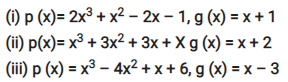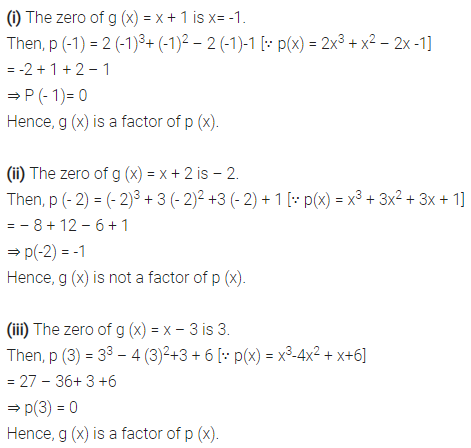# Use the Factor Theorem to determine whether g (x) is a factor of p (x)

Use the Factor Theorem to determine whether g (x) is a factor of p (x) in each of the following cases